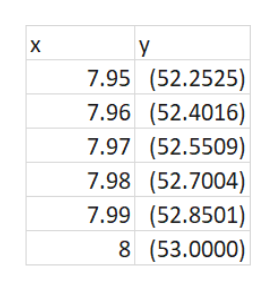# Use the table feature of a graphing calculator to predict the limit. Check your work by using either a graphical or an algebraic approach. (If you need to use  or –, enter INFINITY or –INFINITY, respectively.)lim x→8 f(x), where f(x) =  3 + x − x2    if x≤814 − 9x    if x > 8

Question
1 views

Use the table feature of a graphing calculator to predict the limit. Check your work by using either a graphical or an algebraic approach. (If you need to use  or –, enter INFINITY or –INFINITY, respectively.)

lim x→8 f(x),
where
f(x) =
 3 + x − x2 if x ≤ 8 14 − 9x if x > 8
check_circle

Step 1

Step 1: Go to "Y=", then type in the function 3 + x − x2

Step 2: Go to "TBL SET" (2nd+WINDOW), then set TblStart= 7.95 and ΔTbl=0.01.

You will end up getting the table as shown on the white board.help_outlineImage TranscriptioncloseX y 7.95 (52.2525) 7.96 (52.4016) 7.97 (52.5509) 7.98 (52.7004) 7.99 (52.8501) 8 (53.0000) fullscreen
Step 2

Now repeat the steps for y = 14 − 9x for x > 8

Step 1: Go to "Y=", then type in the function 14 - 9x

Step 2: Go to "TBL SET" (2nd+WINDOW), then set TblStart= 8.01 and ΔTbl=0.01

The output will be as shown on the white board.

...

### Want to see the full answer?

See Solution

#### Want to see this answer and more?

Solutions are written by subject experts who are available 24/7. Questions are typically answered within 1 hour.*

See Solution
*Response times may vary by subject and question.
Tagged in

### Limits